/

### Constrained Quadratic Optimisation: 1. The canonical problem

Next page

1.            The canonical problem

Constrained quadratic optimisation involves finding the value of a vector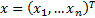that minimises a given penalty function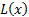(or maximises it, the two are interchangeable by replacing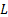with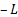) subject to some (normally linear) constraints, where: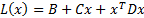The constraints, in canonical form, are normally of two types. There arelower limit constraints of the form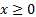(by which we mean that each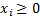for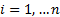) and there are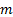further constraints of the form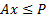(by which we mean that each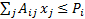for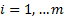).

In these definitions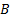is a scalar,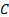is a vector (or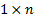matrix) andis an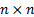symmetric matrix, which is assumed to be non-positive definite if the problem is a minimisation, and non-negative definite if the problem is a maximisation. A non-negative definite (symmetric) matrix is one whose eigenvalues are all at least zero.

The value ofis irrelevant to the optimal value of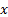so without loss of generality can be taken as zero.

Problems with lower limits on each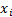that are non-zero, say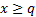can be restated into the above form by a change of variables to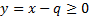resulting in a new penalty function: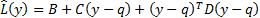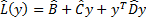Herewhere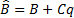,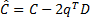and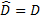. We therefore merely need to alterappropriately, and unwind the change of variables at the end of the optimisation process.

Problems that involve ‘greater than’ or ‘equals’ type constraints, e.g.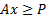or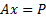as well as (or instead of) ‘less than’ type constraints can be converted into the above canonical form by:

(a)    converting each ‘equality’ constraint into two equivalent constraints, one being the corresponding ‘greater than’ constraint and one being the corresponding ‘less than’ constraint, alteringaccordingly (since ifthenand, and then

(b)   inverting each ‘greater than’ constraint present into a ‘less than’ constraint by noting that if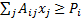then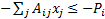.